使用Django管理数据库表

Python版本 :2.7

Django版本: 1.10.5

ORM框架

Django采用ORM模型处理数据库关系编辑models.py文件

vim  monitor/models.py

class oraclelist(models.Model):
port=models.CharField(max_length=50)
tnsname=models.CharField(max_length=100)
version=models.CharField(max_length=100)
charset=models.CharField(max_length=100)
ncharset=models.CharField(max_length=100)
hostname=models.CharField(max_length=100)
content=models.CharField(max_length=300)
monitor_type=models.IntegerField(default=1)
performance_type=models.IntegerField(default=0)
hit_type=models.IntegerField(default=1)
def __unicode__(self):
return self.tnsname
class Meta:
app_label='monitor'
2. content 填写该数据库用途
3. monitor_type,performance_type,hit_type 暂时不介绍，大家照写就行
4. unicode函数中tnsname表示后台显示的时候默认显示tnsname,Python3用str关键字
5. meta 类填写monitor app名称

Django栏位(Field)和原数据库的对用请参考如下连接

同步数据库

python manage.py  makemigrations
python manage.py  migrate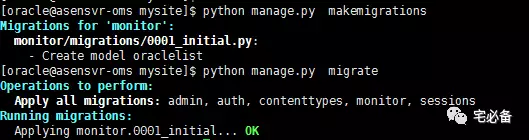from monitor.models import oraclelist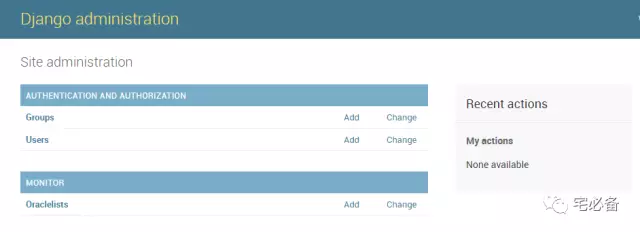填写需要运维的Oracle数据库信息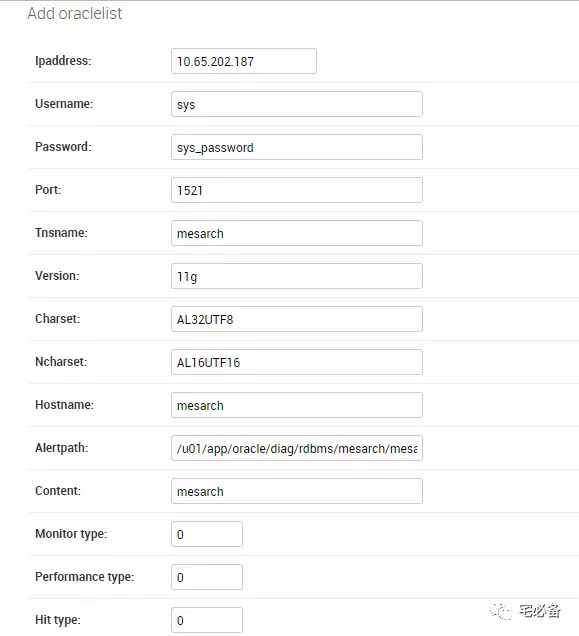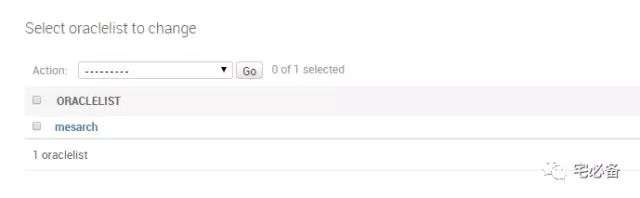修改表结构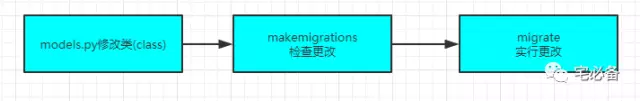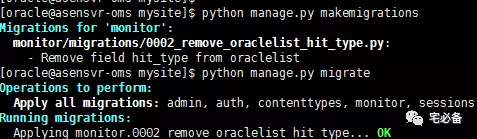https://github.com/bsbforever/wechat_monitor